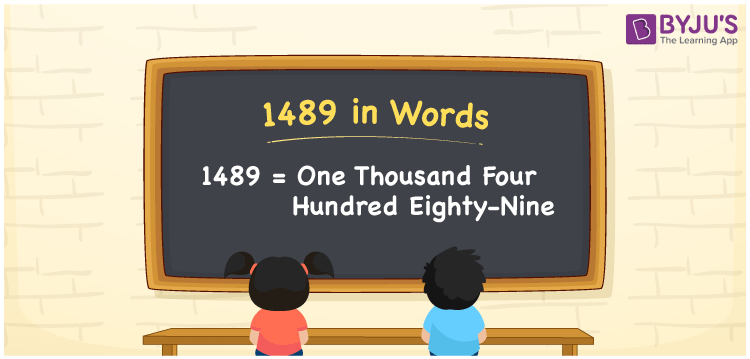# 1489 in Words

The number can be written 1489 in words as One thousand four hundred eighty-nine. If you spent Rs. 1489 for buying a sports shirt, you can say, “I spent One thousand four hundred eighty-nine to buy a sports shirt”. You can learn the method of converting the cardinal number 1489 into words, along with some interesting facts about 1489, here in this article.

 1489 in words One thousand four hundred eighty-nine One thousand four hundred eighty-nine in Numbers 1489

## 1489 in English Words

Using the English alphabet, we generally express numbers in words. So, we spell 1489 in English as “One thousand four hundred eighty-nine”.## How to Write 1489 in Words?

The conversion of 1489 into words is simple when we use the place value chart. So, let’s make a place value chart for 1489, as shown below.

 Thousands Hundreds Tens Ones 1 4 8 9

Here, ones = 9, tens = 8, hundreds = 4, and thousands = 1.

By expanding these numbers, we get;

1 × Thousand + 4 × Hundred + 8 × Ten + 9 × One

= 1 × 1000 + 4 × 100 + 8 × 10 + 9 × 1

= 1000 + 400 + 80 + 9

= One thousand + Four hundred + Eighty + Nine

= One thousand four hundred eighty-nine

Therefore, 1489 in words = One thousand four hundred eighty-nine.

As we know, 1489 is a natural number that precedes 1490 and succeeds 1488.

1489 in words – One thousand four hundred eighty-nine

Is 1489 an even number? – No

Is 1489 an odd number? – Yes

Is 1489 a prime number? – Yes

Is 1489 a composite number? – No

Is 1489 a perfect square number? – No

Is 1489 a perfect cube number? – No

## Frequently Asked Questions on 1489 in Words

Q1

### How do you write 1489 in words?

Generally, we can write 1489 in words as One thousand four hundred eighty-nine.
Q2

### What number is One thousand four hundred eighty-nine in words?

The number 1489 is expressed as “One thousand four hundred eighty-nine” in words.
Q3

### How to write Rs. 1489 in words on a cheque?

On a cheque, an amount of Rs. 1489 can be written in words as “One thousand four hundred eighty-nine rupees only”.